You are not registered yet. Please click here to register!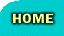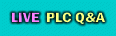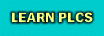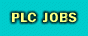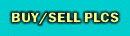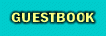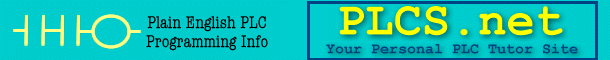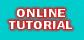This board is for PLC Related Q&A ONLY. Please DON'T use it for advertising, etc.Try our online PLC Simulator- FREE.  Click here now to try it.New Here? Please read this important info!!!PLCS.net - Interactive Q & APID, which one is in your PLC?July 27th, 2004, 03:42 AM #1 Peter Nachtwey MemberJoin Date: Apr 2002 Location: No income tax, no capital gains tax. Freedom! Posts: 8,300 PID, which one is in your PLC? Two spread sheets with different forms of PID The first PID uses only the error for all three terms of the PID. Control engineers would say that all the gains are in the forward path. This is the same PID and system used last week. The second PID is what many call a I-PD. I-PD uses the error only for the I term and it uses only the PV for the P and D terms. In this case only the I gain is in the forward path. The P and D terms are in the feedback path because they only depend on the PV or feedback. Hence the name I-PD. The point of the exercise is to compare the two PIDs and to realize that NO MATTER HOW MUCH YOU TURN UP THE GAINS YOU CAN NOT REDUCE THE ERROR TO ZERO. The PID is much better at following the sine wave and the I-PD is much better at responding to the step changes. This means that you should have the right PID for the right application. If the application uses a target generator with smooth changes to the SP, the PID with all the gains dependent on the error should be used. However, for most temperature systems where the SP is changed in steps, the I-PD form should be used. This spread sheet is not a tuning exercise. The tuning exercises are over for now. You may have noticed that you can get the ITAE or ISE much lower than what is prudent by increasing the gains. In some cases the gains were so hot that they could go unstable if anything in the system changes. The PID has one major flaw because it doesn't provide any output unless there is, or has been, an error. It doesn't make any difference how much to crank up the gains. There is a way to reduce error to almost 0. This technique is called feed forward. I will release a feed forward spread sheet in a day or two. HJuly 27th, 2004, 11:44 AM #2 RussB Lifetime Supporting MemberJoin Date: Oct 2003 Location: Michigan Posts: 2,914 PID, IND or ISA? In a GE Fanuc Series 90-30,70 or VersaMax the programmer must choose which one they need or want as these PLCs have both types of function blocks. PID_IND or PID_ISAJuly 27th, 2004, 12:37 PM #3 kamenges MemberJoin Date: Nov 2002 Location: Brillion, WI Posts: 4,309 Peter- When I opened up the .zip file it had your Type 0, one complex pole pair spreadsheet from last week (T0C1 PID A.zip). Is that the right one? KeithJuly 27th, 2004, 01:01 PM #4 Peter Nachtwey MemberJoin Date: Apr 2002 Location: No income tax, no capital gains tax. Freedom! Posts: 8,300 there are more options that just two So far all my PIDs have been what RussB calls the PID_IND. That is because each gain is independent from the others. The so called ISA equation has only ONE gain that is applied to all three terms of the PID. The integrator and differentiator gains are expressed as time constants but they too are mulitplied by the overall gain. IND OR ISA HAS NOTHING TO DO WITH WHETHER THE DIFFERENTIATOR IS MULTIPLIED BY THE ERROR OR THE PV. I bring this up because few know the difference and yet one can see that the difference can be large IF you have studied the spread sheets. So RussB does the GE PLC apply the differentiator gain to the error or just the PV? What about the Proportional gain? Is the proportional gain mulitplied by the error or the PV? If you look at the equations I use in the PID and I-PD you will see this Code: ```PID: Control(n) = Control(n-1) + Ki*T*e(n) + Kp*(e(n)-e(n-1)) + (Kd/T)*(e(n)-2*e(n-1)+e(n-2)) I-PD: Control(n) = Control(n-1) + Ki*T*e(n) - Kp*(PV(n)-PV(n-1)) - (Kd/T)*(PV(n)-2*PV(n-1)+PV(n-2))``` Notice the difference between the two equations. The I-PD uses only the PV for calculating the P and D terms and notice also the P and D gains have a minus signs in front. How can this be? Remember that e(n) = SP(n) - PV(n) Now lets just look at the differentiator. In the PID form the differentiator is (Kd/T)*(e(n)-2*e(n-1)+e(n-2)) Now substitue SP(n)-PV(n) for e(n) (Kd/T)*((SP(n)-PV(n))-2*(SP(n-1)-PV(n-1))+(SP(n-2)-PV(n-2))) Now the I-PD algorithm works best when SP(n) = SP(n-1) = SP(n-2). In otherwords the SP is not ramped from one set point to another. If all the set points are the same then the above equation simplifies to (Kd/T)*((-PV(n))-2*(-PV(n-1))+(-PV(n-2))) The SP terms cancel out. If I factor out a minus 1 then this term becomes -(Kd/T)*(PV(n)-2*PV(n-1)+PV(n-2)) This now looks like the differentiator term in the I-PD. The I-PD is optimized for systems where the SP does not change except by steps. The PID is best for motion control where the SP is ramped smoothly from one setting to another. The PID reacts violently to step changes.July 27th, 2004, 04:26 PM   #5
rytko
MemberJoin Date: Sep 2002
Location: Beaverton, Oregon
Posts: 56
Quote:
 So RussB does the GE PLC apply the differentiator gain to the error or just the PV?
Peter, this is user-selectable by setting a bit in a configuration word. Straight from the 90-30 Instruction Set Manual:

Quote:
 Derivative = (Error  previous Error)/dt or (PV  previous PV)/dt if 3rd bit of Config Word set to 1.July 28th, 2004, 03:17 AM   #6
Peter Nachtwey
MemberJoin Date: Apr 2002
Location: No income tax, no capital gains tax. Freedom!
Posts: 8,300
Good. GE users have a choice.

Quote:
 Originally posted by rytko Peter, this is user-selectable by setting a bit in a configuration word. Straight from the 90-30 Instruction Set Manual:
Now everyone knows what that bit is for and when it should be used.

Rytko, is there a bit for configuring the proportional gain to act on the error or the PV too.

Rytko, what does setting the bit that switches the P or D gain from acting on the error, to acting on the PV, do to the transfer function? What does it do to the Bode plot? Consider this an extra credit question.July 28th, 2004, 11:04 PM #7 Peter Nachtwey MemberJoin Date: Apr 2002 Location: No income tax, no capital gains tax. Freedom! Posts: 8,300 No one answered Ok, I have a link that shows the difference in response between PID, PI-D and I-PD controlling the last weeks system. I you are afraid of math then skip to the plots at the bottom. Bode Plots for PID, PI-D and I-PD controlling the last weeks system . Ideally the magnitude should be at 0 db and the phase at 0 too. This means the PV is exactly tracking the SP. You can see a higher frequencies the magnitude drops off and the phase goes negative. This shows how the system response is attenuated at higher frequencies and the PV will lag the SP. See the spread sheet T0C1 I-PD. The spread sheets move the SP at 2 hz. Looking at the Bode plots one can see how the PI-D and PID do pretty well because their output is still at about 0 db and not attenuated whereas the I-PD is attenuated about 5 or 6 db so at two hz the PV would follow the SP at only about half the amplitude of the SP. Rytko, the gains in the forward loop at zeros. The zeros add phase lead that compensate for the poles phase lag. This is why the PID keeps the gain flatter than those PIDs that have fewer zeros. It is the zeros that make the system jump in response to noise or step changes in the SP.July 28th, 2004, 11:19 PM #8 kamenges MemberJoin Date: Nov 2002 Location: Brillion, WI Posts: 4,309 Only loosely related... I thought a zero was, by definition, a value of 's' that caused the transfer function to go to zero and a pole was a value of 's' that cause the transfer function to go to infinity. Why is it that the addition of a zero, which should cause attenuation at that location in the frequency spectrum, will produce a phase lead? KeithJuly 29th, 2004, 01:33 AM   #9
Peter Nachtwey
MemberJoin Date: Apr 2002
Location: No income tax, no capital gains tax. Freedom!
Posts: 8,300
Very related. Good question

Quote:
 Originally posted by kamenges I thought a zero was, by definition, a value of 's' that caused the transfer function to go to zero and a pole was a value of 's' that cause the transfer function to go to infinity.
This is correct. This is the mathematicians definition of poles and zeros. [rant] Unfortunately it doesn't give one a good feel for what poles and zeros really do in real applications and this is why there are questions like that below. Professors just repeat what was state above and do make the effort to impart the real knowledge so one has an intuitive feel.[/rant]

Quote:
 Originally posted by kamenges Why is it that the addition of a zero, which should cause attenuation at that location in the frequency spectrum, will produce a phase lead? Keith [/B]
Let's take a simple lead lag transfer function

Code:
```(s+a)
-----
(s+b)```
The s+a part is the lead part with a zero at -a.
The zero will not go to 0 unless s is equal to -a.
Assume a is a positive number like 3.

The s+b part is the lag part with a pole at -b.
The pole will not go to 0 unless s is equal to -b.
Assume b is a positive number like 4.

Fortunatly jw ( w is omega, the frequency in radians ) is substituted for s and omega goes from 0 to infinity. Notice the j for imaginary numbers. Frequencies are represented by imaginary numbers. When omega = 0 and the system is at stead state the transfer function will be just be a/b or 3/4. As omega approaches infinity the gain will approach 1. Infinity + 3 = infinity. Infinity + 4 = infinity. Infinity/infinity = 1. Because the poles are negative the transfer function is well behaved at all frequencies. Fortunately there is not a frequency at -b or the equation would blow up.

When omega = 2 the transfer function is:

Code:
```j2+3
-----
j2+4```
You can see that when omega is 0, the numerator has a magnitude of sqrt(0^2+3^2) = 3 and the phase angle is atan(0/3)=0 degrees.

Now examine the numerator 3+j2. The magnitude of this number is sqrt(2^2+3^2) = 3.6. The phase angle is atan(2/3)=33.6 degrees.
Try different values of omega. As the frequency increases the magnitude and phase angle ( lead ) will increase.

Numerator has a greater magnitude and phase when omega is eqaul to 2 than it does when omega is 0.

Basically you do the same for the denominator.

When you divide the numerator by the denominator, you divide the magnitude of the numerator by the magnitude of the denominator and subtract the phase angle of the denominator from the phase angle of the numerator.

There isn't any value of jw that will cause either the numerator or denominator to go to 0.Currently Active Users Viewing This Thread: 1 (0 members and 1 guests)
 Thread ToolsShow Printable VersionEmail this Page Display ModesLinear ModeSwitch to Hybrid ModeSwitch to Threaded ModePosting Rules You may not post new threads You may not post replies You may not post attachments You may not edit your posts BB code is On Smilies are On [IMG] code is On HTML code is Off Forum Rules
 Forum Jump User Control Panel Private Messages Subscriptions Who's Online Search Forums Forums Home PLCS.net - Interactive Q & A     Announcements     LIVE PLC Questions And Answers     Practice Posting Here     Archives Casual TalkSimilar Topics Thread Thread Starter Forum Replies Last Post tomcoll LIVE PLC Questions And Answers 6 November 13th, 2013 08:15 AM Manuel Raposo LIVE PLC Questions And Answers 22 July 16th, 2007 08:24 AM stooperbike LIVE PLC Questions And Answers 2 November 1st, 2004 10:22 AM qee LIVE PLC Questions And Answers 5 April 9th, 2004 11:59 AM Ron Beaufort LIVE PLC Questions And Answers 11 February 22nd, 2003 12:57 PM

All times are GMT -4. The time now is 08:27 AM.

 -- PLCS.net - Interactive Q & A Normal Style ---- PLCS.net - Interactive Q & A Bigger 10% Style -- PLCS.net - Interactive Q & A Mobile Style PLCS.net - Text - Top

.